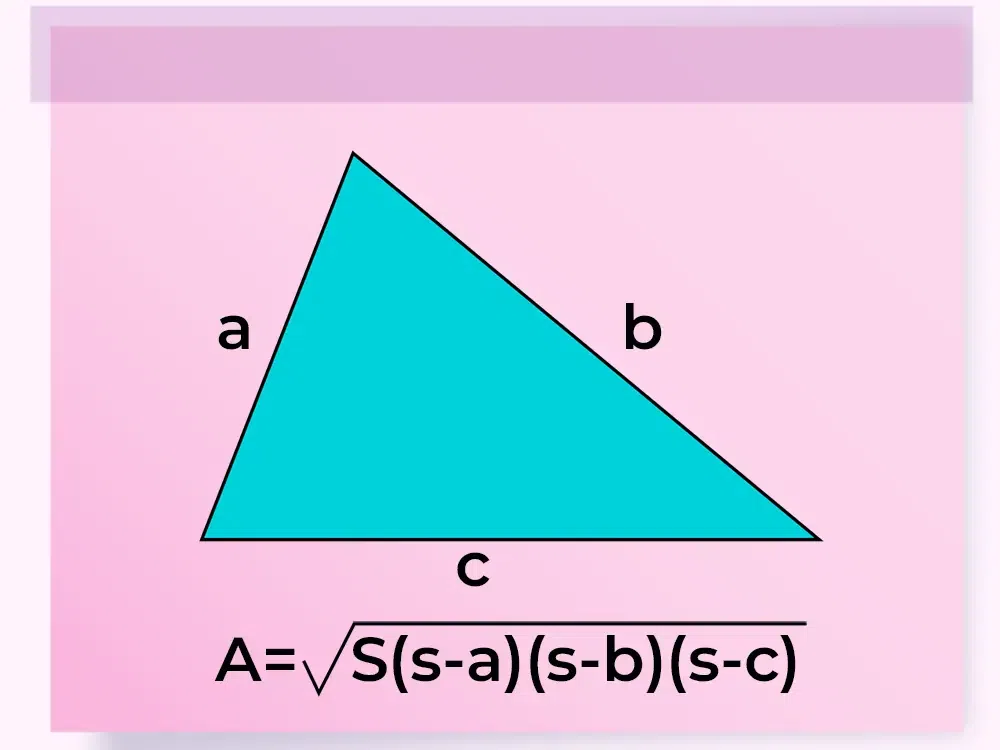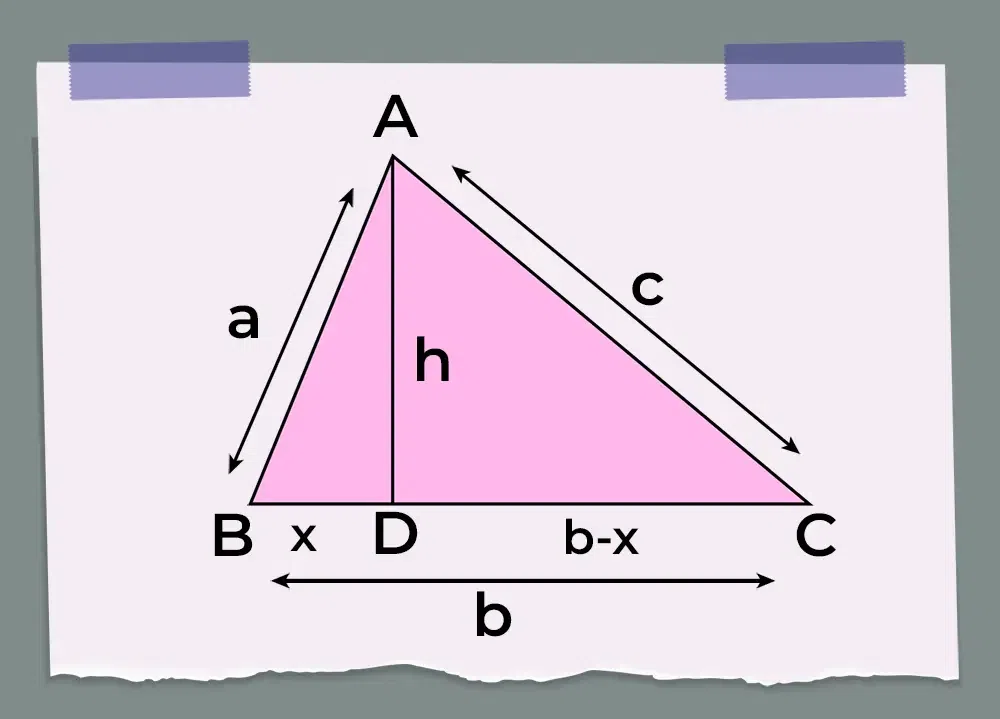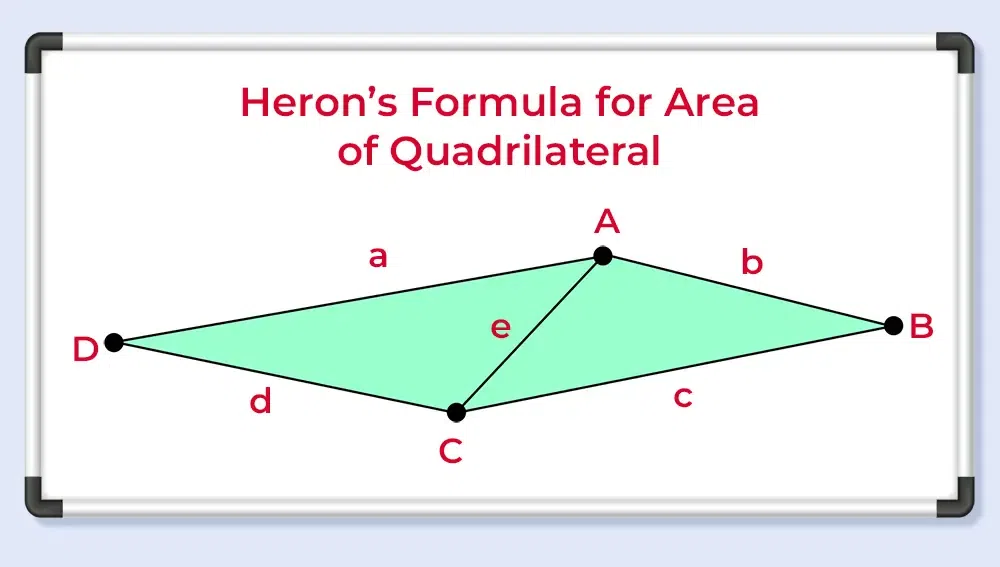GFG App
Open AppBrowser
Continue

Heron’s formula is a very popular formula for finding the area of a triangle when the three sides are given. This formula was given by “Heron” in his book “Metrica”. We can apply this formula to all types of triangles, be they right-angled, equilateral, or isosceles. Let’s learn about Heron’s formula and its derivation in detail.

## What is Heron’s Formula?

Heron’s formula is helpful in determining the area of a triangle if the value of the length of all the sides of the triangle is given. Heron’s formula is also used for finding the area of a quadrilateral as a quadrilateral can easily be divided into two triangles by the diagonals of the quadrilateral. According to this formula, the area of a triangle is directly proportional to the square root of the semi-perimeter of the triangle. If the sides of the triangle are a, b, and c then Heron’s Formula is,

A = √{s(s-a)(s-b)(s-c)}

Where,

• A is area of Triangle ABC,
• a, b, c are lengths of the sides of the triangle, and
• s is semi-perimeter = (a + b + c)/2.### Heron’s Formula Definition

Heron’s formula, states that “For a triangle having lengths, a, b, and c, and semi-perimeter of the triangle is ‘s’ the area of the triangle using Heron’s Formula” is discussed in the image above.

### History of Heron’s Formula

In 60 CE Heron of Alexandria gave a formula which is known as the Heron’s Formula. Heron was a Greek Mathematician who gave the formula for finding the area of a triangle when the length of all sides of the triangle is given. He used this formula for solving various problems in triangles and trigonometry. He proved the Law of Cosine and the Law of Cotangent using this formula.

## Proof for Heron’s Formula

Heron’s formula is derived using Pythagoras Theorem, the Area of a Triangle, and others. For a triangle having lengths of sides, a, b, and c. The semi-perimeter of the triangle ABC is “s”,

s = (a + b + c) / 2

Now Heron’s Formula to calculate the area of the triangle ABC is,

A = √{s(s-a)(s-b)(s-c)}

The derivation of Heron’s Formula

### Heron’s Formula Derivation Using Pythagoras Theorem

The proof for Heron’s Formula is discussed in the article below

Area of a Triangle = (1/2) × b × h

Where,

• b is the base, and
• h is the height.

The image shown below shows the triangle which is braked into two triangles and then we apply Pythagoras theorem to them,Draw a perpendicular AD on BC

From the ∆ ABD,

a2 = x2 + h2

⇒ x2 = (a2−h2)….(i)

⇒ x = √(a2−h2)….(ii)

Consider the  ∆ACD,

(b−x)2 + h2 = c2

⇒ (b−x)2 = c2 − h2

⇒ b2 − 2bx + x2 = c2–h2

Putting the value of x and x2 from equations (i) and (ii) in the above equation, we get

b2 – 2b√(a2−h2)+ a2−h2 = c2 − h2

⇒ b2 + a2 − c2 = 2b√(a2 − h2)

Squaring on both sides, we get;

(b2 + a2 – c2)2 = 4b2(a2−h2)

⇒ {(b2 + a2 – c2)2) / 4b2 = (a2−h2)

⇒ a2 + {(b2 + a2 – c2)2) / 4b2 = h2

simplifying, we get

h2 = (a+b+c)(b+c-a)(a+c-b)(a+b-c) / 4b2

Now, 2s = a+b+c, where s is the semi-perimeter of the triangle.

h2 = 2s(2s-2a)(2s-2b)(2s-2c) / 4b2

⇒ h = √[2s(2s-2a)(2s-2b)(2s-2c)] / 2b

⇒ h = 2×√[s(s-a)(s-b)(s-c)] / b…(iii)

From, area of triangle = 1/2 × b × h

Now, area of triangle = 1/2 × {b × 2×√[s(s-a)(s-b)(s-c)]} / b

Area of Triangle (A) = √[s(s-a)(s-b)(s-c)]

### Heron’s Formula Derivation Using Cosine Rule

Heron’s Formula can also be easily solved using the Cosine Rule. Now for any triangle ABC if the sides of the triangle are a, b, and c and their opposite angles are, α, β, and γ.

The law of cosine states,

cos γ = (a2 + b2 – c2)/2ab

cos2 γ + sin2 γ = 1

⇒ sin γ = √(1 – cos2 γ)

⇒ sin γ = √[1 – {(a2 + b2 – c2)/2ab}2]

⇒ sin γ = √[(4a2b2 – (a2 + b2 +c2)2]/2ab

If the base of the triangle is a then its altitude is b sin γ

Area of Triangle = 1/2 base ×height

⇒ Area of Triangle = 1/2 × a × b sin γ

⇒ Area of Triangle = 1/2 ab × √[(4a2b2 – (a2 + b2 +c2)2]/2ab

⇒ Area of Triangle = 1/4 √[(a+b)2 – c2]

⇒ Area of Triangle = √(b+c-a)(a+c-b)(a+b-c)(a+b+c)/16

Area of Triangle = √s(s-a)(s-a)(s-b)

Where s = (a+b+c)/2 is the semi perimeter.

## How to Find Area of Triangle Using Heron’s Formula?

Follow the following steps to find the area of a triangle using Heron’s formula

Step 1: Calculate the perimeter of the given triangle

Step 2: Divide the value of the perimeter by 2 to get the semi-perimeter of the given triangle; S = (a+b+c)/2

Step 3: Use Heron’s formula A = √(s(s – a)(s – b)(s – c) to find the area of the triangle.

Thus, the area of the triangle is found.

## Heron’s Formula for Equilateral Triangle

For an equilateral triangle, all sides are equal. Now, the semi-perimeter of the equilateral triangle is

s = (a+a+a) / 2

⇒ s = 3a / 2

where a is the length of the side.

Now, using Heron’s Formula,

Area of Equilateral Triangle = √(s(s – a)(s – a)(s – a)

Area of Equilateral Triangle = √3 / 4 × a2

## Heron’s Formula for Scalene Triangle

As we already know that all the sides in the scalene triangle are different, i.e. no two sides are equal in the scalene triangle. We can easily find the area of the scalene triangle using Heron’s formula. For any triangle ABC where sides AB = a, BC = b and CA = c we can find its area using,

Area of scalene triangle ABC(A) = √s(s-a)(s-b)(s-c)

Where s is the semi-perimeter i.e., s = (a + b + c)/2.

## Heron’s Formula for Isosceles Triangle

Isosceles Triangle is a triangle that has two equal sides, their area can be easily calculated using Heron’s Formula. For any isosceles triangle, ABC where sides AB = a, and BC = a are equal and the third side is CA = b. The formula for its area is,

Area of iscosceles triangle ABC(A) = √s(s-a)(s-a)(s-b)

Where s is the semi-perimeter i.e., s = (a + b + c)/2.

Simplifying, A = √s(s-a)(s-a)(s-b)

⇒ s = a + b/2

⇒ A = √s(s-a)(s-a)(s-b)

⇒ A = √(a + b/2)(b/2)(b/2)(a – b/2)

⇒ A = √(a + b/2)(a – b/2)(b/2)(b/2)

A = √[(a2 – b2/4)(b2/4)]

## Heron’s Formula for Area of Quadrilateral

Heron’s formula is used to determine the formula for the area of the quadrilateral. We can divide the quadrilateral into two separate triangles using any one of its diagonals and then the area of the two separate triangles is calculated using theHeron’s Formula.

The area of the quad ABCD is calculated by dividing it into two triangles using its diagonal. Let’s say we join the vertices A and C to form the diagonal AC then we divide it into two triangles △ABC and △ADC. If we take the length of sides of the quadrilateral as,

AB = b, BC = c, CD = d, and DA = a, and the length of diagonal AC is e then its area is calculated using,Area of quad ABCD = Area of △ABC + Area of △ADC…(i)

Area of triangle ABC

Area of △ ABC = √(s1(s1 – b)(s1 – c)(s1 – e))

Where s1 = (b + c + e)/2.

Area of △ ADC = √(s2(s2 – d)(s2 – a)(s2 – e))

Where s2 = (d + a + e)/2.

Thus,

form eq(i)

Area of quad ABCD = √(s1(s1 – b)(s1 – c)(s1 – e)) + √(s2(s2 – d)(s2 – a)(s2 – e))

Where,

• s1 = (a + b + e)/2, and
• s2 = (a + d + e)/2

## Applications of Heron’s Formula

Heron’s formula is various applications and some of the important applications of Heron’s Formula are,

• For finding the area of the triangle if the sides of the triangle are given
• For finding the area of the quadrilateral if the length of all the sides and the length of the diagonal are given.
• For finding the area of any polygon if its sides and the length of all the principal diagonals are given.

### Area of Polygon using Heron’s Formula

We can also find the area of the polygon using Heron’s Formula. A polygon is a figure formed by joining n straight lines. Its area is calculated using Heron’s Formula by using the steps discussed below,

Step 1: Make sure all the sides of the polygon are given.

Step 2: Divide the n-sided polygon into n-1 triangles and mark the length of all the triangles.

Step 3: Find the area of each triangle using the Herons Formula

Step 4: Add the areas of all the triangles to get the required area of the polygon.

Also, Check

## Solved Examples on Heron’s Formula

Example 1: Calculate the area of a triangle whose lengths of sides a, b, and c are 14cm,13cm, and 15 cm respectively.

Solution:

Given:

a = 14cm
b = 13cm
c = 15cm

Firstly, we will determine semi-perimeter(s)

s = (a + b + c)/2

⇒ s = (14 + 13 + 15)/2
⇒ s = 21 cm

Thus, A = √(s(s – a)(s – a)(s – a)

⇒ A = √(21(21 – 14)(21 – 13)(21 – 15)

⇒ A = 84 cm2

Example 2: Find the area of the triangle if the length of two sides is 11cm and 13cm and the perimeter is 32cm.

Solution:

Let a, b and c be the three sides of the triangle.

a = 11cm
b= 13 cm

c = ?

Perimeter = 32cm

As we know, Perimeter equals to the sum of the length of three sides of a triangle.

Perimeter = (a + b + c)

⇒ 32 = 11 + 13 + c

⇒ c = 32 – 24

⇒ c= 8 cm

Now as we already know the value of perimeter,

s = perimeter / 2

⇒ s = 32 / 2

⇒ s =16 cm

As, a = 11cm, b = 13 cm, c = 8 cm, s = 16 cm

Thus,  A = √(s(s – a)(s – a)(s – a)

⇒ A = √(16(16 – 11)(16 – 13)(16 – 8)

⇒ A = 43.8 cm2

Example 3: Find the area of an equilateral triangle with a side of 8 cm.

Solution:

Given,

Side = 8 cm

Area of Equilateral Triangle = √3 / 4 × a2

⇒ Area of Equilateral Triangle = √3 / 4 × (8)2

⇒ Area of Equilateral Triangle = 16 √3 cm2

## FAQs on Heron’s Formula

### Q1: What is Heron’s Formula?

Heron’s Formula is the formula to find the area of a triangle when its three sides are given,

A = √{s(s-a)(s-b)(s-c)}

### Q2: Who gave Heron’s formula?

Heron’s formula was given by Hero of Alexandria a great mathematician of ancient Greece.

### Q3: What does ‘s’ represent in Heron’s Formula?

‘s’ in Heron’s formula represents the semi-perimeter of the triangle which is calculated as,

s = (a+b+c)/2

where,
a, b and c are three sides of a triangle.

### Q4: When is Heron’s formula used?

We use Heron’s formula for finding the side of the triangle when all its three sides are known.

### Q5: What is Heron’s formula for Equilateral Triangle?

Area of an equilateral triangle with side ‘a’ can be found using the formula,

A = √3 / 4 × a2